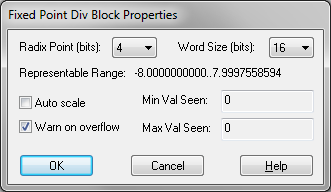## greater than or equal to (>=)Block Category: Boolean

Inputs: Real, complex, or fixed-point scalars, or vectors or matrices.

l: numerator x1.

r: denominator x2.

Description: The >= block generates an output signal of 1 if and only if input signal x1 is greater than or equal to input signal x2. Otherwise, the output is 0.

Right-click the >= block to assign a different function to the block.

#### Example

1. Simple if-then-else construct

Consider a variable y such that:

If x ≥ 0.5 then y = sin(t); else y = 1

where t is simulation time and x is a unit step delayed by 3 sec. This is usually represented as u(t - 3). This system is realized as:Until the onset of the step input at t = 3 sec, the Boolean inequality x 0.5 evaluates to false and y takes on a value of 1. At t = 3 sec, the Boolean inequality evaluates to true and remains true for the duration of the simulation duration. Consequently, from this point onwards, y takes on the value of sin(t).